## 21.5 More on the Infinite Continued Fraction Series

### A. The Difference between consecutive members of the F Series

We have discovered that our F&D Series’ approach the square roots of the rational numbers. How do they approach it? More specifically how does our F Series, with the D Series at its base, approach the square roots?

#### Posing some questions

Do the elements of the F Series get bigger and bigger with each iteration – approaching the Limit from below? Or do they get smaller and smaller approaching the Limit from above? Or do they bounce around the Limit in some inexplicably weird pattern? What happens to the relative value of the members of the F Series with each iteration? To understand the progression of F Series elements towards their Limit let us begin this exploration by examining the difference between consecutive members of the F Series.

#### ƒ(N) - ƒ(N-1) = ?

Using results from above we come up with the following equations after simplifying algebraically.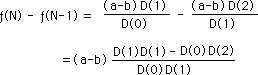Let us analyze our result intuitively for perspective. The 'a-b' term is fixed. D(0)D(1) is always positive when a/b > 1. The only ambiguous term is the numerator. We would guess that the numerator probably approaches zero as N -> ∞ pushing the difference to zero, meaning that the successive elements would get closer and closer together and to their Limit.

#### Sub-question: D(0)D(0) - D(-1)D(1) = d(N)2- d(N+1)d(N-1) =? = g(N)

However intuition needs to be grounded in the reality of facts and/or deductive reasoning in order to have any substance. This leads us to our next question. What does the numerator equal? Or more specifically, what is the difference between the square of a member of the D Series and the product of the two adjacent members? Let g(N) = our difference. What is g(N)?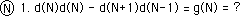The circled number in front represents the case we are testing. We predict intuitively that g(N) approaches 0 as N approaches infinity.

#### Cases when N = 1, 2, 3, 4 & 5

Let us work our way through some individual cases. We know that d(1) = 1 and that d(0) = 0, therefore g(1) = 1.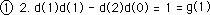For the case when N is 2, we expand d(3) in the general style. Simplifying we get that g(2) = -K, the scaling factor.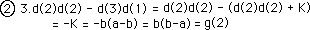For the case when N =3, we first expand one of the d(3)s and d(4) in the normal way. The first and third terms are equal and have opposite signs so their sum equals zero. We factor out a K from the remaining terms. This leaves us with -g(2) inside the parenthesis. Simplifying we find that g(3) = (-K)^2.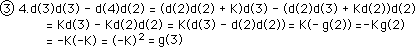Similarly for the case when N = 4, we expand one of the d(4)s and d(5). Again our first and third terms are equal and opposite, canceling each other out. We factor a K from the remaining terms and inside the parenthesis is -g(3). Substituting and simplifying we find g(4). A pattern is emerging.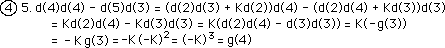To find g(5), we expand d(5) and d(6). Surprise of surprises, our first and third terms are equal and opposite canceling each other out. We factor a -K out of the remaining terms leaving g(4) inside the parenthesis. Substituting and simplifying we find g(5).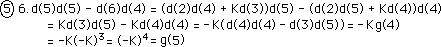#### General Case: d(N)2- d(N+1)d(N-1) = (-K)N-1

Finally to find g(N) we expand d(N) and d(N+1). As expected the first and third terms cancel. We factor a -K out of the remaining terms. This leaves g(N-1). Logical gap. Extending our intuitive logic we have g(N).#### Conclusions

There are two conclusions from the above derivation. First g(N) alternates between negative and positive with each iteration, because N, the number of iterations, is in the exponent. Second the absolute value of g(N) ->∞ as N->∞ rather than approaching zero as we thought it would.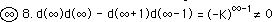So much for intuition; thank god for deduction and experimental facts.

#### How does that work?

What does this mean for our initial question? First let us substitute the above result into our question equation at the beginning of the section. The difference of consecutive members of the F Series becomes: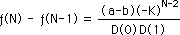From earlier computer experimentation we know that the F Series approaches a distinct Limit. Because of this we know that the difference between consecutive F Series elements must approach zero. How can the difference approach zero when the numerator alternates between positive and negative while its absolute value grow larger and larger? We must have done something wrong. This doesn’t make sense.

#### Thank god for Computer testing

Let us do a computer experiment to verify or falsify our derivations. Breaking the values of the numerator and denominator of the above equation apart, we see that their absolute values both get increasingly larger. (See Appendix.) This is in line with our derivations. However we also see that their ratio, which is the F Series Difference, approaches zero, which is also what we expected. Ironically while the numerator of the above fraction which equals g(N) approaches infinity as N approaches infinity, the denominator D(0)D(1), or d(N)d(N-1), approaches infinity so much faster that the ratio of numerator to denominator approaches zero. This is a case of where infinity over infinity equals zero.

#### A Computer anomaly

This experiment also yielded an interesting computer anomaly. Our value for g(N) grew larger and larger with each iteration and then suddenly turned zero for g(20) when a/b = 2. Disturbing. The reason was that while the difference between d(20)d(20) and d(21)d(19) was huge that the difference relative to their individual values was beyond the computer’s 16-place accuracy. In other words the first 16 digits of both numbers were identical with the entire huge difference occurring in the digits after these first 16. The huge difference exceeded the precision of computer. (See appendix for results.)

#### F Series brackets its Limit

This explains one of the features of our F Series Difference Formula, i.e. that the absolute value of the numerator grows larger and larger. The second feature was that the numerator, and hence the fraction representing the Difference, alternated between positive and negative. In terms of consecutive differences, our difference is positive, then negative, then positive again. These differences alternate signs because of the (-K)^(N-2) factor. When N is even our difference is positive making ƒ(N) > ƒ(N-1). When N is odd our difference is negative making ƒ(N) < ƒ(N-1). We are speaking about the condition when a/b > 1. Simultaneously the Difference approaches zero. Thus our F series elements must bracket their Limit going a little over and then a little under.

#### A Specific example: when a/b = 2

Let us look at a specific example. Let a = 2 and b =1. Then K = 1. Here is our specific equation for the differences.Notice that our numerator is always 1 or -1. Hence its magnitude is the same. The sign only reflects the bracketing of the square root. Each element of the F series is alternately higher then lower than the square root of two. While the absolute magnitude of the numerator is always equal, the denominator grows as N grows. Hence the absolute value of the difference between consecutive members of our F Series shrinks as N grows larger. Furthermore because of the alternation of greater and less than, there is really no exact place that our limit is approaching. It is a little higher or a little lower. In this case the little bit is always +1 or -1. The numerator does not approach zero. The denominator grows larger and larger and hence the fraction grows smaller and smaller. The fraction approaches zero because of the denominator but no matter how great N is the numerator always alternates between 1 and -1.

#### Implications: An infinity of points between each number

Because the numerator does not approach zero and, in fact, only alternates signs, it does not disappear as N becomes larger. Hence our limit exists in theory but not in reality. No matter how great N is our square root function has that pesky one to deal with. It is always a little greater or a little lesser. The one never disappears, hence the reality of the limit disappears. Because of this vibration that doesn't disappear, there are an infinity of points between every number. No matter how great N is, there will be a '-1' in the numerator followed by a positive '1'.

#### Different from a fractional decimal series

This differs from a fraction represented in decimal fashion, whose Limit can exist, mentally at least. For instance 1/3 is created by adding 3/10 to 3/100 to 3/1000 to 3/10000 ad infinitum. While we have to keep adding 3 to the decimal there is no pesky one in the way to always send us over or under our Limit no matter how many times we iterate. Decimals always approach their distinct Limit from below giving the illusion that the Limit is just ahead but something real nevertheless. Here the absolute limit seems to exist. With infinite continued fractions, the numerator is overwhelmed in size by the denominator, but can’t shrink away to nothing.

#### Even iterations greater than odd iterations: ƒ(Even) > ƒ(Odd)

For further clarification let us look at the difference between the even and odd iterations. Analyzing our F Series Difference equation from above, we find these results.Summarizing our results we see that ƒ(Even) > ƒ(Odd) for all even and odd manifestations of the functions. Hence our functions bounce about the square root of two without ever hitting it. The even functions are a little greater than the square root while the odd functions are a little smaller than the square root. There is no point that is the square root. Hence comes the concept that there is an infinity of points between each point in the infinite continued fraction system.#### Between even and odd or never there, always balancing

The positive to negative vibration is endless. ‘There’ doesn't exist, only the alternation, the approximation. It is possible to get as close as desired to the goal, but it is impossible to get there, because ‘there’ is in the middle between the even and the odd. What is between even and odd? The sound of one hand clapping, of course. One never arrives, as soon as you get close to your mark you overshoot and must return, always overshooting by less and less each time but never arriving at the Promised Land, because it doesn't exist. The Yin always follows the Yang, which follows the Yin. Endlessly. Only the Tao is in the middle and it is Nameless and Unknowable.

#### Pure Number is an illusion, an approximation, existing between even and odd.

Under this scheme, number becomes what it always was, an approximate magnitude. Even pure number when examined closely evaporates into an approximation. Number does not exist exactly but only as an approximation. It is as exact as necessary but its reality is between even and odd, because that is the only place in-between.

#### Numbers exist in an impossible place

Here we are, looking at ourselves in a mirror, reflecting upon the infinite mysteries of Creation. We look at a number, a simple number, and find nothing there. As part of the larger more inclusive square root family, numbers are characterized by the iterative F series, which bounces endlessly around the number, approximating it to any desired accuracy, while never reaching it. The Number and Limit of this series exists between even and odd, a paradoxically impossible place. This is a metaphor for Reality, which is based in the impossible place of Being between our polar Thoughts.

#### D Series has a tight inner structure

The F Series for square roots is itself a function of a D series. This D series has many interesting properties. In the first manifestation of the D Series, i.e. d(N) = 2b d(N-1) + K d(N-2), the two previous elements were crucial in determining the next member of the series. (We saw that the geometric series is a special case of the D Series when only one element is needed to generate the next. This happens only when the Root Number, a/b. equals one.) In analyzing our series a little further, we found that the 2b d(N-1) term could be written as d(2)d(N-1) which could further be written as d((N+1)) = d(R)d(N-R+1). Similarly we found that the second term, K d(N-2), could be generalized to read K d((N-1)) = K d(R-1)d(N-R). Thus the Nth term of our series is actually based upon an intricate interlocking of four previous members of the series. The simplest manifestation has D Series based upon the two previous elements, but the internal structure of the D series is so intense that this is only a surface manifestation. Although countless variations of the four members are possible, once one of the elements is determined all the rest immediately follow. The self-reflective quality of the D Series for square roots yields an intense crystalline structure influenced only slightly by birthplace, i.e. seeds.

### B. The Raveling of the F&D Series

In the past section we examined how our F Series approached the Root by looking at the difference between consecutive F Series values. In this section we will examine how our F Series approaches its Limit by looking even more carefully at the Denominator series. We will discover that the elements of the D Series can be raveled up into a power series. This raveling connects the D Series with our Data Streams Derivatives developed in previous Notebooks. It also assists us in understanding the construction of the F Series and its approach to its Limit.

#### Derivation: Pre-Raveling

Let us start with the generalized description of the D series.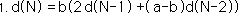Let us shift notation for economy and clarity. As we did in the Seed Equation Notebook, we call N, the Now, and R, the level Removed from Now. This inverts our reference from zero to N. This is signified by the capital D rather than the small D in the function. We’ve already shown this notation in this Notebook.Rewriting the equation in the Remainder style, then dividing through by b, the denominator of our rational square root, we get this.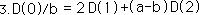Using Equation 1 on D(2).A further notational simplification for clarity and economy. Note the use of K again as a constant. We've chosen it because it will turn into a scaling factor like it was in the Seed Equation Notebook.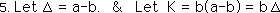Rewriting Equation 1, the defining equation of our D series with the new notational abbreviations.Remember that the delta symbol is a constant representing the difference between the numerator and the denominator of our rational square root. It does not represent the difference between consecutive elements of the D series.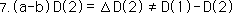Writing equation 4 with the new notation.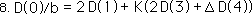Using equation 6 on D(4) and substituting into equation 8, we get.We then multiply through by ∆, remembering that ∆*b = K. We then factor out a 2.First we factor out a K from the right half of the equation. We then duplicate the same process we employed in steps 9 and 10. We use equation 6 on D(6) and multiply through by ∆.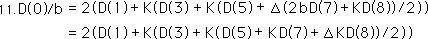We now divide both sides of our equation by 2, for more simplification.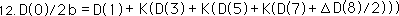We multiply through by the Ks to remove the last parentheses.We employ the same substitution from Equation 4 on D(8) this time in the last term of the expansion.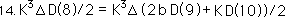We multiply through by ∆ and factor out the K as before.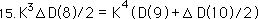We now substitute this expansion into equation 13.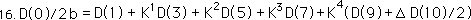#### Quickie General Proof

A quickie general proof of the same process. First substitute Equation 4 for D(R), then multiply through by ∆, factor out a K. We then begin again using this process on D(R+2). It is easily seen that this process could be repeated ad infinitum, successively substituting for D(R+4), D(R+6), ... Practically speaking however our D series begins with d(0). See derivation above. D(N) = d(0). Hence the process need only be used until D(N) is reached.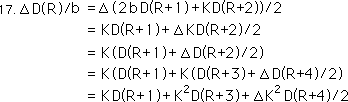With the above information, we can write Equation 16 as a power series.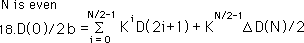Multiplying through by 2b.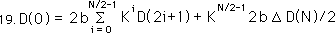Simplifying.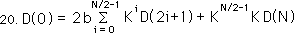Combining Ks in the last term of the expression.Remembering certain definitions.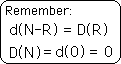When N is even, the last term drops out because D(N)=0.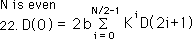Going back to equation 18 and starting with N-1, when N is odd.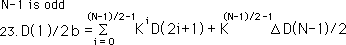Multiplying through by 2b.Simplifying.Remembering that D(N-1) = 1.Simplifying equation 24, we get the general expression when the value inside the parentheses is odd.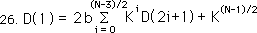#### General expression

Below are the two expressions that can expand d(N) if N is odd or even.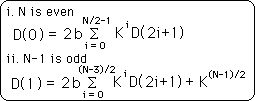Now let us look at how this intensified sense of structure is reflected in the F Series whose limit determines the square roots.

#### Derivation: A new look at the F Series

For simplification let us create a new type of F Series that is just the ratio of consecutive members of the D series. (Note that this F(N) is in no way connected with the F(0) = ƒ(N). Sorry about the confusion. If edited this would be changed.)The initial member of this series is undefined because d(0) = 0 and is in the denominator.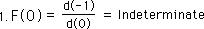The first member is equal to zero because d(0) is in the numerator.Applying the above definitions we get 1/2b for F(2).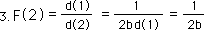We then apply the same definitions to the next few members of the series. For F(3) we see an 'extra' K in the denominator. Also a different way of writing it.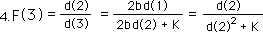For F(4) we see an 'extra' K in the numerator.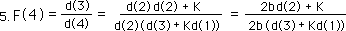For F(5) we see an 'extra' K^2 in the denominator.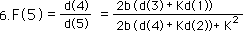For F(6) we see an 'extra' K^2 in the numerator.For F(7) we see an 'extra' K^3 in the denominator. A pattern is beginning to emerge.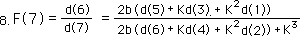#### Raveling at last! A Notational Shift

Let us introduce another raveling pattern to simplify our notational mess. This just rewrites the summation that we saw above in a simpler form. We write an even and an odd form in order to facilitate understanding.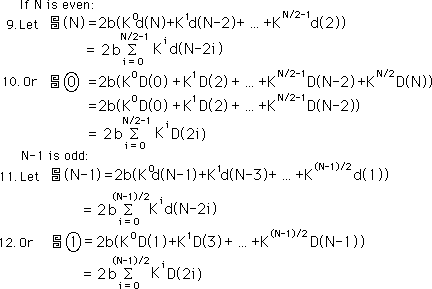#### Back to the structural breakdown of our F Series

With F(8) we write our expression in both forms. With this even expression the unraveled, 'extra' K^3 comes in the numerator this time.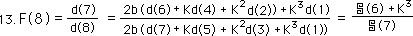With F(9) the unraveled K^4 is in the denominator.With F(10) the unraveled K^4 is in the numerator. The pattern becomes clearer.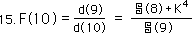#### A Summary of the Patterns

When N is even, d(N) can be expressed as a raveled number with no extra K term on the end. However when N is odd then the extra K term is added on the end as a sum to the unraveling. The bottom expression is the same expression in Remainder notation.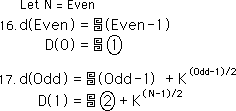We can now write our F function in simplified terms. When N is even it can be written as follows – taking into account the definitions laid out above. One can easily see that the extra K term always ends up in the numerator. The equation below is the same expression in Remainder notation.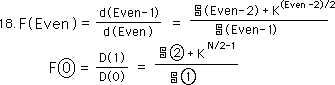When N is odd we get the following generalization. Notice that the extra K term is now in the denominator.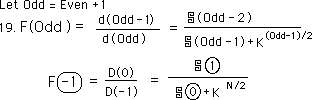#### Some inequalities

It is easy to see that F(Even) is greater than the raveled expression on the right without the K expression in the numerator.It is also easy to see that F(Odd) is less than the raveled expression on the right without the K expression in the denominator.#### A Mini-number line analysis: some observations.

F(Odd) is at the lower left end of our mini-number line. F(Even) is at the higher right end. Sandwiched between are the ratios of consecutive raveled numbers. As F(Even) and F(Odd) both approach the promised land the raveled ratios between are caught in the middle.#### Unconfirmed speculations

As N becomes larger the K term shrinks to insignificance against the raveled number next to it. Hence the ratio of consecutive raveled terms approaches each other. (This has not been proved.)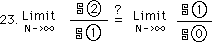Hence we can represent the inequality below.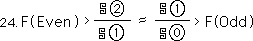Reconverting back to the original F Series we can make the following generalizations, which confirm our previous results. Additionally we see that when a/b < 1 that ƒ(Even) becomes the smaller and ƒ(Odd) the larger, reversing the relationships when a/b > 1. This is because the K term becomes negative when a/b < 1 because b>a and a-b is negative.#### Summary: the Limit is an illusion

In the examination of the approach of the F Series to its Limit, we first looked at the difference between consecutive elements of the F Series. In this section we examined the internal structure of the D Series. In so doing we were able to ravel it up. We then applied these ravelings to the F Series. In the clarity of raveling we saw once again how the Limit is an illusion. Once again there is a pesky KN that won’t go away. It is either in the numerator if N is even or in the denominator if N is odd. Further it grows larger with each iteration, not smaller. However its growth is more than neutralized by the raveled D Series, which is a type of power series. While its growth is neutralized this term never goes away, meaning that the Limit still exists between the even and odd iterations, which is a point that doesn’t exist in this world. In the next section we will delve even deeper into this F Series approach to its Limit, discovering imaginary lines and complex spirals in the process. We don’t know how it happens. Read on to discover how Forms imperfectly reflect Reality.